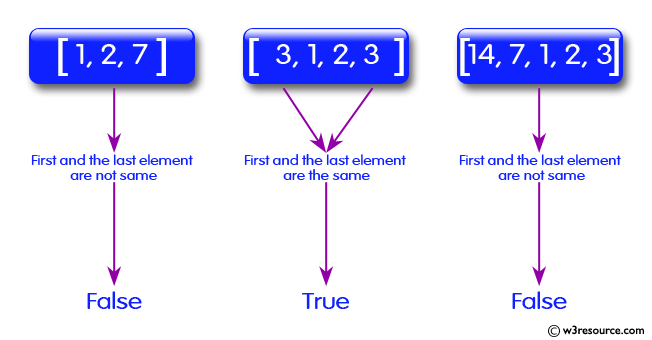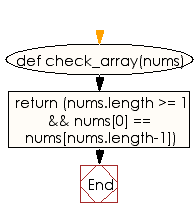﻿ Ruby Array exercises: Check whether first and the last element are the same of a given array of integers - w3resource# Ruby Array Exercises: Check whether first and the last element are the same of a given array of integers

## Ruby Array: Exercise-4 with Solution

Write a Ruby program to check whether first and the last element are the same of a given array of integers. The array length must be 1 or more.Ruby Code:

``````def check_array(nums)
return (nums.length >= 1 && nums ==  nums[nums.length-1])
end
print check_array([1, 2, 7]),"\n"
print check_array([3, 1, 2, 3]),"\n"
print check_array([14, 7, 1, 2, 3])
``````

Output:

```false
true
false
```

Flowchart:Ruby Code Editor: